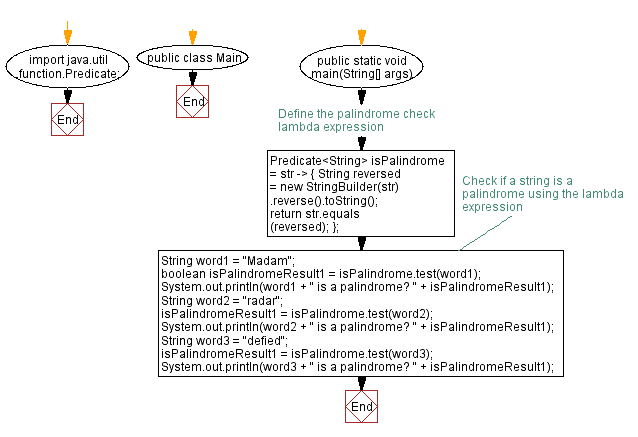# Java program to check Palindrome string using lambda expression

## Java Lambda Program: Exercise-14 with Solution

Write a Java program to implement a lambda expression to check if a given string is a palindrome.

Sample Solution:

Java Code:

``````import java.util.function.Predicate;

public class Main {
public static void main(String[] args) {
// Define the palindrome check lambda expression
Predicate < String > isPalindrome = str -> {
String reversed = new StringBuilder(str).reverse().toString();
return str.equals(reversed);
};

// Check if a string is a palindrome using the lambda expression
boolean isPalindromeResult1 = isPalindrome.test(word1);
System.out.println(word1 + " is a palindrome? " + isPalindromeResult1);

isPalindromeResult1 = isPalindrome.test(word2);
System.out.println(word2 + " is a palindrome? " + isPalindromeResult1);

String word3 = "defied";
isPalindromeResult1 = isPalindrome.test(word3);
System.out.println(word3 + " is a palindrome? " + isPalindromeResult1);
}
}
``````

Sample Output:

```Madam is a palindrome? false
defied is a palindrome? false

```

Explanation:

In the above exercise -

• From the java.util.function package, we import Predicate.
• In the main method, we define a lambda expression str -> { ... } to check if a string is a palindrome.
• Inside the lambda expression, we create a reversed version of the input string by using a StringBuilder to reverse the characters. We convert it back to a string using toString().
• Compare the original string with the reversed string using the equals() method. Return true if they are equal, indicating that the string is a palindrome. Otherwise, it returns false.
• Create an instance of the Predicate interface called isPalindrome and assign the lambda expression to it.
• Test the lambda expression by checking some strings are palindromes or not using the test() method on the isPalindrome predicate.

Flowchart:Live Demo:

Java Code Editor:

Improve this sample solution and post your code through Disqus

Java Lambda Exercises Previous: Java Program to count words in a sentence using lambda expression.
Java Lambda Exercises Next: Java program to calculate sum of squares of odd and even numbers using lambda expression.

What is the difficulty level of this exercise?

Test your Programming skills with w3resource's quiz.

﻿